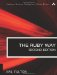# Section 5.25. Finding the Mean, Median, and Mode of a Data Set

### 5.24. Finding Logarithms with Arbitrary Bases

When working with logarithms, we frequently use the natural logarithms (or base e, sometimes written ln); we may also use the common or base 10 logarithms. These are defined in Ruby as Math.log and Math.log10, respectively.

In computer science, specifically in such areas as coding and information theory, a base 2 log is not unusual. For example, this will tell the minimum number of bits needed to store a number. We define this function here as log2:

`def log2(x)   Math.log(x)/Math.log(2) end`

The inverse is obviously 2**x just as the inverse of log x is Math::E**x or Math.exp(x).

Furthermore, this same technique can be extended to any base. In the unlikely event that you ever need a base 7 logarithm, this will do the trick.

`def log7(x)   Math.log(x)/Math.log(7) end`

In practice, the denominator should be calculated once and kept around as a constant.The Ruby Way, Second Edition: Solutions and Techniques in Ruby Programming (2nd Edition)
ISBN: 0672328844
EAN: 2147483647
Year: 2004
Pages: 269
Authors: Hal Fulton

Similar book on Amazon